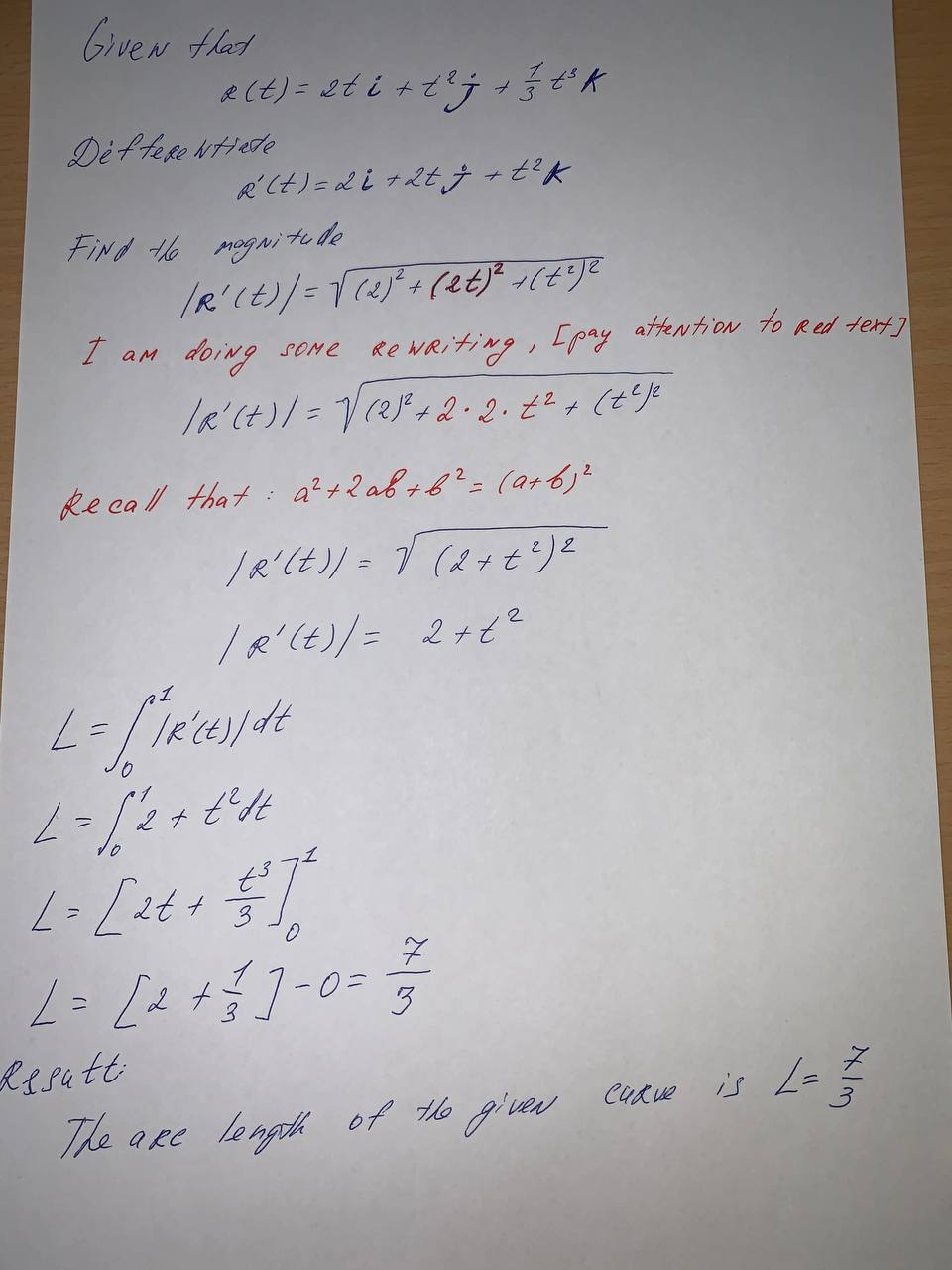# Find the length of the curve. r(t)=<2t, t^2, \frac{1}{3}t^3> 0\leq t\leq1hexacordoK 2021-09-18 Answered
Find the length of the curve.
$r\left(t\right)=<2t,{t}^{2},\frac{1}{3}{t}^{3}>$
$0\le t\le 1$
You can still ask an expert for help

• Questions are typically answered in as fast as 30 minutes

Solve your problem for the price of one coffee

• Math expert for every subject
• Pay only if we can solve itClara Reese

We have to find the length of the given curve.